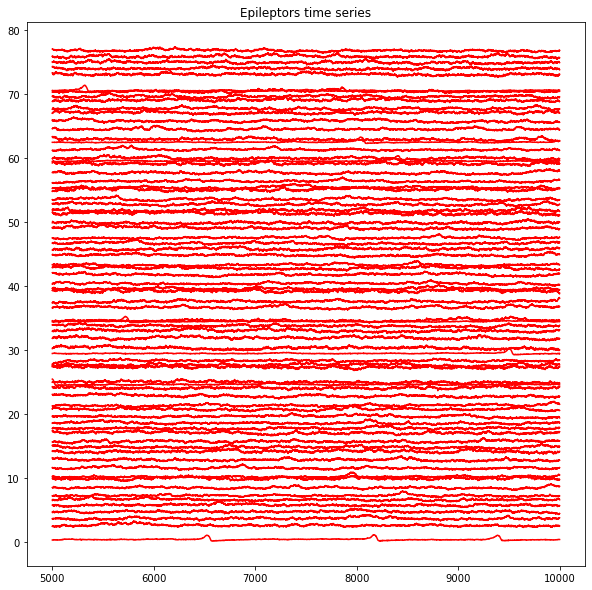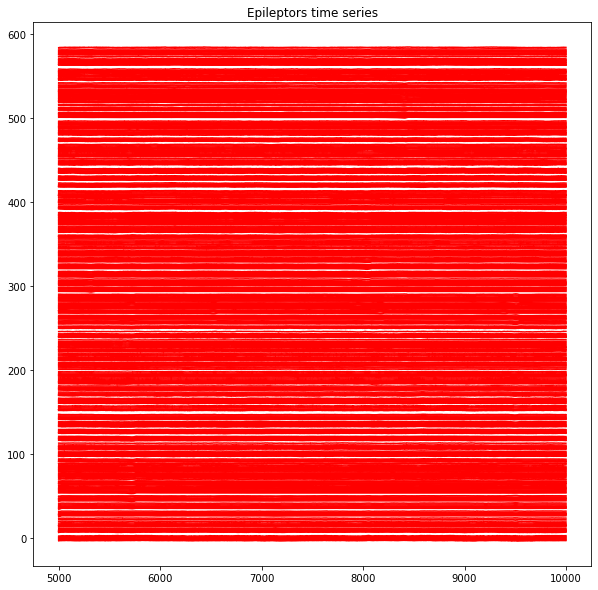In :
from tvb.simulator.lab import *

import os.path
from matplotlib import colors, cm
import numpy as np
import time
import scipy.signal as sig
import scipy.io as sio

import matplotlib.pyplot as plt
import seaborn as sns


# Set Directories To Look For Metadata¶

To simulate the TVB, you will need the connectivity data for this patient.

In :
connectivity_path = 'connectivity_76.zip'


# Initialize Connectivities, Model, Coupling, Integrators and Monitors¶

Initialize the core parts of the TVB simulator and then simulate

In :
con = connectivity.Connectivity.from_file(connectivity_path)
con.speed = np.array([sys.float_info.max])                      #set conduction speed (here we neglect it)
con.cortical[:] = True     # To avoid adding analytical gain matrix for subcortical sources
# normalize
con.weights = con.weights/np.max(con.weights)
nb_regions = len(con.region_labels)

WARNING  File 'hemispheres' not found in ZIP.

In :
x0ez=-1.6
x0pz=-2.4
x0num=-2.4
period=1.

ezind = 0

coupl = coupling.Difference(a=np.array([1.]))

#Initialise some Monitors with period in physical time
mon_tavg = monitors.TemporalAverage(period=1.)

print(nb_regions)

# Integrator
hiss = noise.Additive(nsig = np.array([0.001, 0.001, 0., 0.0001, 0.0001, 0.]))
heunint = integrators.HeunStochastic(dt=0.05, noise=hiss)

# Epileptor model
epileptors = models.Epileptor(Ks=np.array([-2]), r=np.array([0.0002]), tau = np.array(), tt = np.array([0.07]))
epileptors.x0 = -2.4*np.ones(nb_regions)
epileptors.x0[ezind] = -1.6

epileptors.state_variable_range['x1'] = np.r_[-0.5, 0.1]
epileptors.state_variable_range['z'] = np.r_[3.5,3.7]
epileptors.state_variable_range['y1'] = np.r_[-0.1,1]
epileptors.state_variable_range['x2'] = np.r_[-2.,0.]
epileptors.state_variable_range['y2'] = np.r_[0.,2.]
epileptors.state_variable_range['g'] = np.r_[-1.,1.]

76


Here, you have options for adding observation noise into the monitor level. The normal way for creating a monitor for the SEEG signals would be to maybe read in the:

1. seeg xyz positions from a file
2. gain matrix from a file (e.g. from a dipole, mean-field computation)

Then you would normally set different keyword arguments for the monitor, such as period of the signal you want to sample. Here, we can also include a keyword argument called obsnoise, which we can intialize from the noise module.

In :
# adding observation noise?
ntau=0 # color of noise?
noise_cov=np.array([1.0]) # cov of noise

# monitors
mon_tavg = monitors.TemporalAverage(period=1.0)
mon_SEEG = monitors.iEEG.from_file(sensors_fname='seeg_588.txt',
projection_fname='projection_seeg_588_surface_16k.npy',
period=period,
variables_of_interest=np.array(),
obsnoise=obsnoise
)

num_contacts = mon_SEEG.sensors.labels.size

# run simulation
sim = simulator.Simulator(model=epileptors,
connectivity=con,
coupling=coupl,
conduction_speed=np.inf,
integrator=heunint,
monitors=[mon_tavg, mon_SEEG])

sim.configure()

(ttavg, tavg), (tseeg, seeg) = sim.run(simulation_length=10000)

   INFO  Projection configured gain shape (588, 76)

In :
# Normalize the time series to have nice plots
tavgn = tavg/(np.max(tavg, 0) - np.min(tavg, 0))
seegn = seeg/(np.max(seeg, 0) - np.min(seeg, 0))
seegn = seegn - np.mean(seegn, 0)

# remove the first 5 seconds of data (from initial conditions) for prettier plotting
ttavg = ttavg[5000:]
tavg = tavg[5000:,...]
seeg = seeg[5000:,...]

In :
# Normalize the time series to have nice plots
tavg /= (np.max(tavg,0) - np.min(tavg,0 ))

#Plot raw time series
plt.figure(figsize=(10,10))
plt.plot(ttavg[:], tavg[:, 0, :, 0] + np.r_[:con.number_of_regions], 'r')
plt.title("Epileptors time series")

#Show them
plt.show()In :
# Normalize the time series to have nice plots
seeg /= (np.max(seeg,0) - np.min(seeg,0 ))

#Plot raw time series
plt.figure(figsize=(10,10))
plt.plot(ttavg[:], seeg[:, 0, :, 0] + np.r_[:seeg.shape], 'r')
plt.title("Epileptors time series")

#Show them
plt.show()